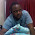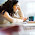## Sunday, 30 October 2011

### Modelling with R: part 5

In our exercise of learning modelling in R, we have till now succeeded in doing the following:

Specifically, we created a decision tree using the rpart package. The decision tree was built with no priors and gave an AUC value of 0.73475 as opposed to 0.80493 given by the logistic regression. So here the logistic regression outperforms the recursive partitioning methodology of the rpart package. In this post we will try to see how we can improve over the current performance level of our decision tree. As a way to gauge our performance in the future, we can consider the AUC of the logistic regression as a minimum benchmark over which we need to improve. So let's get started.

## 2.2.2: Decision tree with priors  ##

t1.prior <- rpart(default ~
amt.fac +
age.fac +
duration +
chk_acct +
history +
purpose +
sav_acct +
employment +
install_rate +
pstatus +
other_debtor +
time_resid +
property +
other_install +
housing +
other_credits +
job +
num_depend +
telephone +
foreign
, data = dev, parms = list(prior = c(0.9, 0.1)))
# Not the difference in the commands for a tree with priors and a tree without one. Here we need to specify the priors along with the formula in the rpart() function command.

Before we go further it is important to understand what "priors" exactly are. I initially did not understand the concept of priors until I read this document. You need not go through the entire document. I have made a little summary for myself that might help.

Simply put, priors are nothing but a weighing scheme. They specify the weight that we put on the overall level of the dependent variable. Technically, all trees are built with priors. In cases where priors are not specified, they are automatically taken to be proportional response rate, i.e, for each class they are proportional to the to the number of records in that class.

Take for example our data set. The dependent variable has two categories - 0 and 1. There are 300 1s and 700 0s, so the response rate is about 30% (300/(700 +300)). Then most decision trees will automatically apply priors of 30% and 70% to the respective classes. Now a simple decision tree tries to maximize the number of cases it classifies correctly and hence a lot of effort will concentrated on classifying the non-defaulters instead of the defaulters. This is because even if we incorrectly classify all the bad loans we will be wrong 30% of the time as opposed to 70% of time if we incorrectly classify the non-defaulters. As mentioned in the document, it is important to note that such a model will be, "literally accurate but practically worthless."

How can we then deal with such situations. There are three possible options here
1. Increase the proportion of defaulters by copying the observations so that there are 700 instances of each.
• Duplicating observations, however, is not a very neat idea.
• Additionally, it can be a problem if the data set is large. Adding observations will only make the other process computationally intensive and/or plain inefficient.
2. Randomly pick 300 non-defaulters and create a data set which has 300 instances of each.
• This involves throwing out a large chuck data that contains valuable information and hence the results may not be accurate
3. Use a smarter decision tree algorithm that let's you specify priors.
In case you haven't already guessed we have taken the third option. If we can vary the priors based on our understanding, we can analyze data without any special handling, regardless of how skewed the distribution of the dependent variable may be.

Coming back to our code
plot(t1.prior)
# Plots the trees
text(t1.prior)
# Adds the labels to the trees.

We don't need to prune this model and can score it right away
val\$t1.p.yhat <- predict(t1.prior, val, type = "prob")

We can plot the ROC curve for the tree with priors.
t1.p.scores <- prediction(val\$t1.p.yhat[,2], val\$default)
t1.p.perf <- performance(t1.p.scores, "tpr", "fpr")

# Plot the ROC curve
plot(t1.p.perf, col = "blue", lwd = 1)

# Add the diagonal line and the ROC curve of the logistic model, ROC curve of the tree without priors
plot(m1.perf, col = "red", lwd = 1, add = TRUE)
plot(t1.perf, col = "green", lwd = 1.5, add = TRUE)
abline(0, 1, lty = 8, col = "grey")
legend("bottomright", legend = c("tree w/o prior", "tree with prior", "logit"), col = c("green", "blue", "red"), lwd = c(1.5, 1, 1))

KS statistic
ks1.p.tree <- max(attr(t1.p.perf, "y.values")[] -(attr(t1.p.perf, "x.values")[]))
ks1.p.tree

AUC
t1.p.auc <- performance(t1.p.scores, "auc")
t1.p.auc

1.Hi MK,

This series of your blog was awesome!!!
It is a perfect tutorial on Modelling in R, compact and concise and very good. I did not get any errors from part 1 to part 5 - very rare, and I did all in one setting consisting of two plus hours.

Thanks again for sharing.

Regards,
KR

1.same here.
I couldn't believe it after all the errors i got from other tutorials.

2.Hi MK,
This is a really nice series !!
I was wondering Can We apply SVM here? Till Now logistics is coming as one of the powerful method. Both the decision tree model had failed here. Can you cover more classification models please. I am following you from last couple of years and you are doing a commendable job. Respect. It is very helpful for us. Thank god and thanks to you.

3.We are private lending firm,We offer Loans at low interest rate of to any Interested Individual personal. We are governmental Registered authorized financial helper. for more information on how to obtain our Loans contact us today via email: (mrhamdnloanoffer@gmail.com)

4.Thank you for sharing! It is really worth sharing and I'm glad that it helped me get Personal Loan Without Documents from Early salary app & it is great.

5.